The MCAT CARS Strategy Course begins February 27!

### Geometrical Optics

Written by Ryan on Jan 5, 2020

## The Ray Aspect of Light

Rays, or beams of light, can travel in three ways: directly from a source, through a material, or indirectly by reflection.

### LEARNING OBJECTIVES

Distinguish three ways that rays can travel

### KEY TAKEAWAYS

#### Key Points

• Direct light motion is when a ray of light travels from a source and is uninterrupted until it reaches it destination.
• Refracted light is when a light ray travels through another medium and, due to the difference in refractive indexes of the materials, changes direction slightly.
• When a light ray hits a reflective material, it bounces off as a reflected ray at a specific angle.

#### Key Terms

• refraction: Changing of a light ray’s direction when it passes through variations in matter.
• geometric optics: Optics that describes light propagation in terms of “rays”.
• reflection: the property of a propagated wave being thrown back from a surface (such as a mirror)

Rays, or beams of light, can travel in three ways: directly, through a material, or indirectly ( reflection ). These three methods of light travel are shown in this image. The word ray comes from mathematics, and refers to a straight line that originates at some point. Even when passing through a material, or bouncing off of a material in a reflection, the light continues to travel in a straight line, even if that line has changed direction. The movement of light, as a ray, can be shown with simple geometry and trigonometry. This is called geometric optics.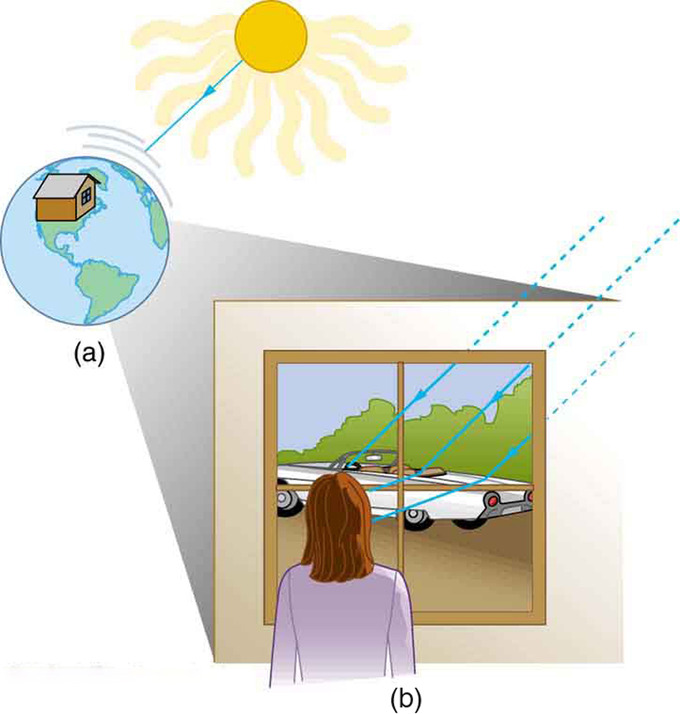Methods of Travel by a Ray of Light: Light can travel through empty space directly from the source, through media like air and glass, reflect from an object like a mirror, or travel in a straight line.

### Direct Light Travel

Direct light travel is when a ray of light starts at a source, and continues to travel from that source to its destination without encountering any interference. The light will continue in a straight line or ray until it reaches the observer. An example of this is the light that travels from the sun to the earth.

### Light Travel Through a Material

When light travels through any material, air, glass, water, etc., it encounters interference and changes direction. This is only a directional change and will continue in this new path, but still as a straight line, or ray. The law that deals with this change in direction is called the law of refraction. This change in ray direction will depend on the refractive index of the material through which the light is travelling. This concept is what led to the development of lenses and glasses. Have you ever noticed that if you put part of a pencil, spoon, or straw in a bowl of water, the object no longer appears straight, but seems to bend? This is because the index of refraction of the water is different from that of the air. This causes the light rays to change direction.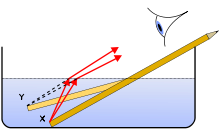Refraction of Light Rays: The concept of refraction explains how a pencil submerged in water appears to bend.

### Light Bouncing Off a Material

When light is bounced off of a material, such as a mirror, this is called a reflection. This is when a light ray, the incident ray, hits a reflective material and bounces off as the reflected ray at a specific angle. This is called the angle of reflection. Since the movement of the light rays can be shown geometrically, if a mirror is one-half your height, you could see your whole body in the reflection.Reflected Rays: This diagram shows how light rays reflects off of a surface.

## The Law of Reflection and Its Consequences

The law of reflection states that the angle of reflection equals the angle of incidence.

### LEARNING OBJECTIVES

Formulate the relationship between the angle of reflection and the angle of incidence

### KEY TAKEAWAYS

#### Key Points

• Light strikes different parts of a rough surface at different angles and is reflected, or diffused, in many different directions.
• A mirror has a smooth surface (compared with the wavelength of light) and so reflects light at specific angles.
• We see the light reflected off a mirror coming from a direction determined by the law of reflection.

#### Key Terms

• reflection: the property of a propagated wave being thrown back from a surface (such as a mirror)

Whenever you look into a mirror or squint at sunlight glinting off a lake, you are seeing a reflection. When you look at the text in a book, you are actually seeing the light that is reflected from it. Large telescopes use reflections to form images of stars and other astronomical objects. In fact, the only way we can see an object that does not itself emit light is if that object reflects light.

The law of reflection is illustrated in, which also shows how the angles are measured relative to the perpendicular to the surface at the point where the light ray strikes. The law of reflection is very simple: The angle of reflection equals the angle of incidence. When we see our reflection in a mirror, it appears that our image is actually behind the mirror — we see the light coming from a direction determined by the law of reflection. The angles are such that our image appears exactly the same distance behind the mirror as we stand away from the mirror.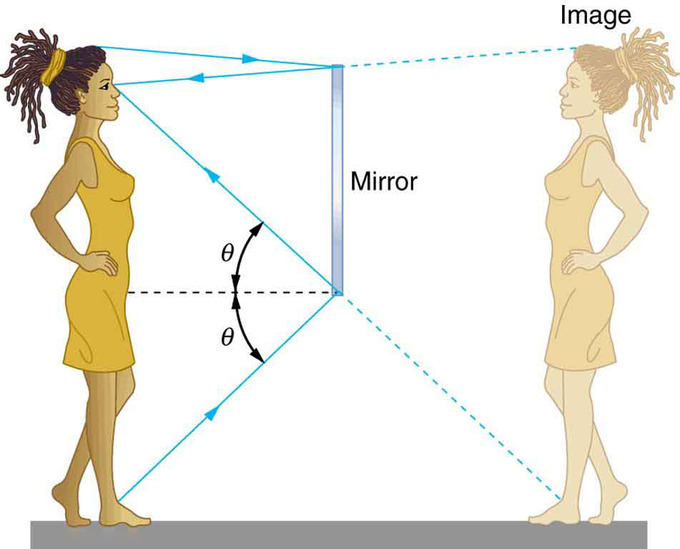Mirror Reflection: An image in a mirror appears as though it is behind the mirror. The two rays shown are those that strike the mirror at just the correct angles to be reflected into the eyes of the viewer. The image appears to come from the direction the rays are coming from when they enter the viewer’s eyes.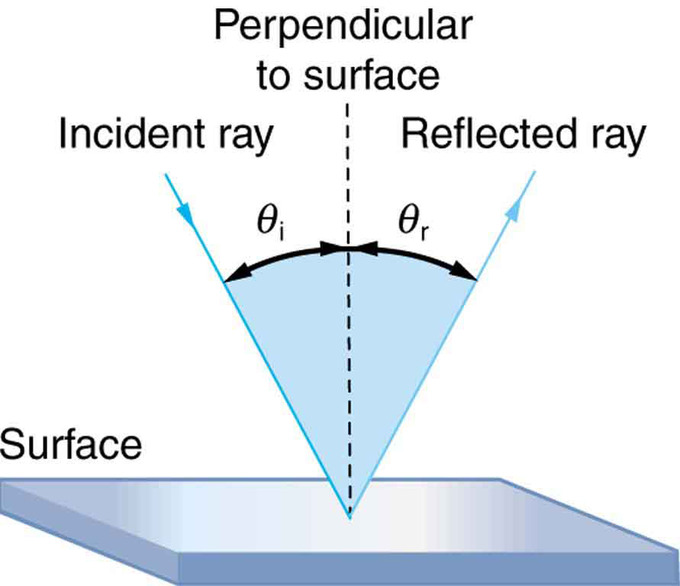Law of Reflection: The law of reflection states that the angle of reflection equals the angle of incidence: θr = θi. The angles are measured relative to the perpendicular to the surface at the point where the ray strikes the surface.

We expect to see reflections off a smooth surface. However, light strikes different parts of a rough surface at different angles, and it is reflected in many different directions (“diffused”). Diffused light is what allows us to see a sheet of paper from any angle. Many objects, such as people, clothing, leaves, and walls, have rough surfaces and can be seen from all sides. A mirror, on the other hand, has a smooth surface (compared with the wavelength of light) and reflects light at specific angles. When the moon reflects off the surface of a lake, a combination of these effects takes place.

Reflection: A brief overview of reflection and the law of reflection.

## The Law of Refraction: Snell’s Law and the Index of Refraction

The amount that a light ray changes its direction depends both on the incident angle and the amount that the speed changes.

### LEARNING OBJECTIVES

Formulate the relationship between the index of refraction and the speed of light

### KEY TAKEAWAYS

#### Key Points

• The changing of a light ray’s direction (loosely called bending) when it passes through variations in matter is called refraction.
• The index of refraction is n=c/v, where v is the speed of light in the material, c is the speed of light in vacuum, and n is the index of refraction.
• Snell’s law, the law of refraction, is stated in equation form as
${\text{n}}_{1}\mathrm{sin}{\theta }_{1}={\text{n}}_{2}\mathrm{sin}{\theta }_{2}$

.

#### Key Terms

• refraction: Changing of a light ray’s direction when it passes through variations in matter.
• index of refraction: For a material, the ratio of the speed of light in vacuum to that in the material.

It is easy to notice some odd things when looking into a fish tank. For example, you may see the same fish appearing to be in two different places. This is because light coming from the fish to us changes direction when it leaves the tank, and in this case, it can travel two different paths to get to our eyes. The changing of a light ray’s direction (loosely called bending) when it passes through variations in matter is called refraction. Refraction is responsible for a tremendous range of optical phenomena, from the action of lenses to voice transmission through optical fibers.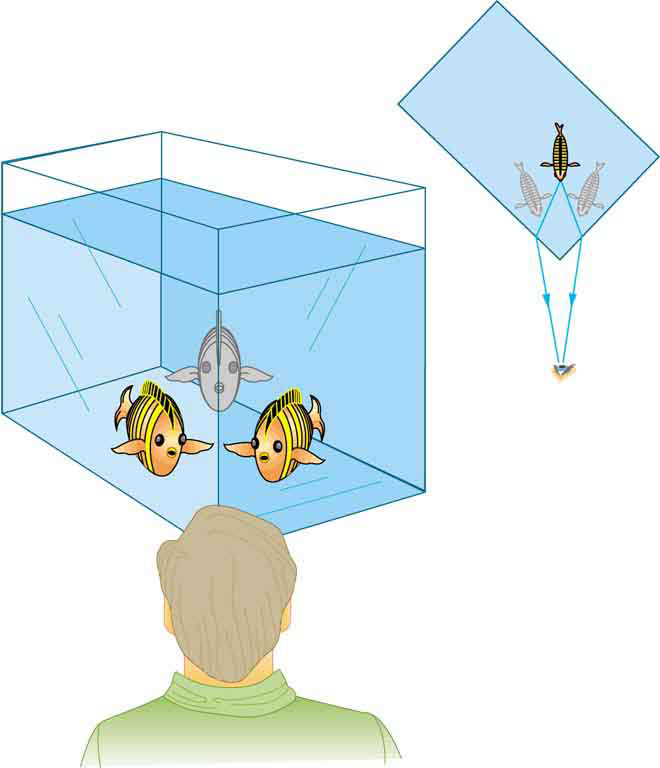Law of Refraction: Looking at the fish tank as shown, we can see the same fish in two different locations, because light changes directions when it passes from water to air. In this case, the light can reach the observer by two different paths, and so the fish seems to be in two different places. This bending of light is called refraction and is responsible for many optical phenomena.

Refraction: The changing of a light ray’s direction (loosely called bending) when it passes through variations in matter is called refraction.

### Speed of Light

The speed of light c not only affects refraction, it is one of the central concepts of Einstein’s theory of relativity. The speed of light varies in a precise manner with the material it traverses. It makes connections between space and time and alters our expectations that all observers measure the same time for the same event, for example. The speed of light is so important that its value in a vacuum is one of the most fundamental constants in nature as well as being one of the four fundamental SI units.

Why does light change direction when passing from one material ( medium ) to another? It is because light changes speed when going from one material to another.

### Law of Refraction

A ray of light changes direction when it passes from one medium to another. As before, the angles are measured relative to a perpendicular to the surface at the point where the light ray crosses it. The change in direction of the light ray depends on how the speed of light changes. The change in the speed of light is related to the indices of refraction of the media involved. In mediums that have a greater index of refraction the speed of light is less. Imagine moving your hand through the air and then moving it through a body of water. It is more difficult to move your hand through the water, and thus your hand slows down if you are applying the same amount of force. Similarly, light travels slower when moving through mediums that have higher indices of refraction.

The amount that a light ray changes its direction depends both on the incident angle and the amount that the speed changes. For a ray at a given incident angle, a large change in speed causes a large change in direction, and thus a large change in angle. The exact mathematical relationship is the law of refraction, or “Snell’s Law,” which is stated in equation form as:

n1sinθ1 = n2sinθ2

Here n1 and n2 are the indices of refraction for medium 1 and 2, and θ1 and θ2 are the angles between the rays and the perpendicular in medium 1 and 2. The incoming ray is called the incident ray and the outgoing ray the refracted ray, and the associated angles the incident angle and the refracted angle. The law of refraction is also called Snell’s law after the Dutch mathematician Willebrord Snell, who discovered it in 1621. Snell’s experiments showed that the law of refraction was obeyed and that a characteristic index of refraction n could be assigned to a given medium.

Understanding Snell’s Law with the Index of Refraction: This video introduces refraction with Snell’s Law and the index of refraction.The second video discusses total internal reflection (TIR) in detail. http://www.youtube.com/watch?v=fvrvqm3Erzk

## Total Internal Reflection and Fiber Optics

Total internal reflection happens when a propagating wave strikes a medium boundary at an angle larger than a particular critical angle.

### LEARNING OBJECTIVES

Formulate conditions required for the total internal reflection

### KEY TAKEAWAYS

#### Key Points

• The critical angle is the angle of incidence above which total internal reflection occurs and given as
${\theta }_{\text{c}}=\mathrm{arcsin}\left(\frac{{\text{n}}_{2}}{{\text{n}}_{1}}\right)$

.

• The critical angle is only defined when n2/n1 is less than 1.
• If light is incident on an optical fiber with an angle of incidence greater than the critical angle then the light will remain trapped inside the glass strand. Light can travel over a very long distance without a significant loss.

#### Key Terms

• Snell’s law: A formula used to describe the relationship between the angles of incidence and refraction.
• cladding: One or more layers of materials of lower refractive index, in intimate contact with a core material of higher refractive index.

Total internal reflection is a phenomenon that happens when a propagating wave strikes a medium boundary at an angle larger than a particular critical angle with respect to the normal to the surface. If the refractive index is lower on the other side of the boundary and the incident angle is greater than the critical angle, the wave cannot pass through and is entirely reflected. The critical angle is the angle of incidence above which the total internal reflectance occurs.

What is Total Internal Reflection?: Describes the concept of total internal reflection, derives the equation for the critical angle and shows one example.

### Critical angle

The critical angle is the angle of incidence above which total internal reflection occurs. The angle of incidence is measured with respect to the normal at the refractive boundary (see diagram illustrating Snell’s law ). Consider a light ray passing from glass into air. The light emanating from the interface is bent towards the glass. When the incident angle is increased sufficiently, the transmitted angle (in air) reaches 90 degrees. It is at this point no light is transmitted into air. The critical angle

${\theta }_{\text{c}}$

is given by Snell’s law,

${\text{n}}_{1}\mathrm{sin}{\theta }_{1}={\text{n}}_{2}\mathrm{sin}{\theta }_{2}$

. Here, n1 and n2 are refractive indices of the media, and

${\theta }_{1}$

and

${\theta }_{2}$

are angles of incidence and refraction, respectively. To find the critical angle, we find the value for

${\theta }_{1}$

when

${\theta }_{2}$

= 90° and thus

$\mathrm{sin}{\theta }_{2}=1$

. The resulting value of

${\theta }_{1}$

is equal to the critical angle

${\theta }_{\text{c}}={\theta }_{1}=\mathrm{arcsin}\left(\frac{{\text{n}}_{2}}{{\text{n}}_{1}}\right)$

. So the critical angle is only defined when n2/n1 is less than 1.Fig 1: Refraction of light at the interface between two media, including total internal reflection.

### Optical Fiber

Total internal reflection is a powerful tool since it can be used to confine light. One of the most common applications of total internal reflection is in fibre optics. An optical fibre is a thin, transparent fibre, usually made of glass or plastic, for transmitting light. The construction of a single optical fibre is shown in.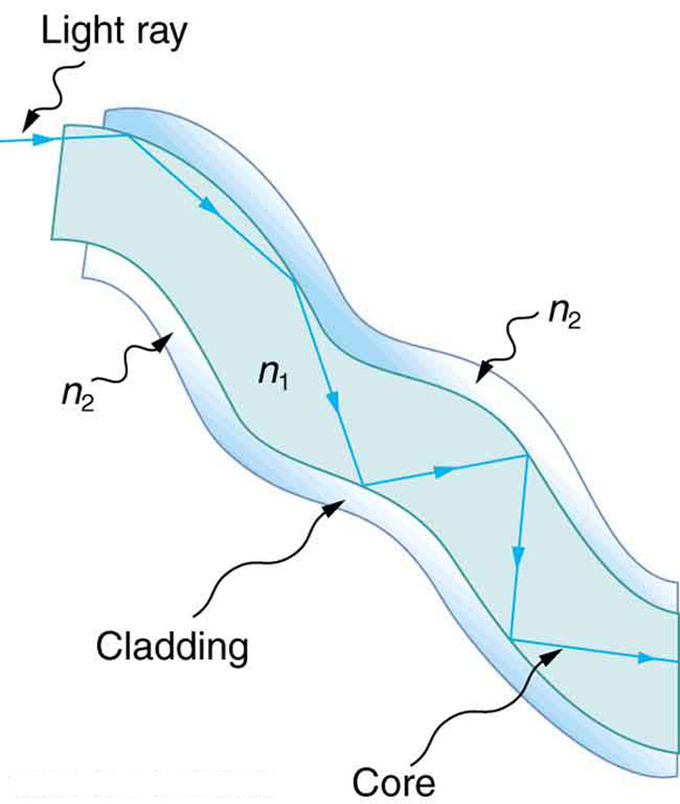Fig 2: Fibers in bundles are clad by a material that has a lower index of refraction than the core to ensure total internal reflection, even when fibers are in contact with one another. This shows a single fiber with its cladding.

The basic functional structure of an optical fiber consists of an outer protective cladding and an inner core through which light pulses travel. The overall diameter of the fiber is about 125 μm and that of the core is just about 50 μm. The difference in refractive index of the cladding and the core allows total internal reflection in the same way as happens at an air-water surface show in. If light is incident on a cable end with an angle of incidence greater than the critical angle then the light will remain trapped inside the glass strand. In this way, light travels very quickly down the length of the cable over a very long distance (tens of kilometers). Optical fibers are commonly used in telecommunications, because information can be transported over long distances, with minimal loss of data. Another common use can be found in medicine in endoscopes. The field of applied science and engineering concerned with the design and application of optical fibers are called fiber optics.

## Total Polarization

Brewster’s angle is an angle of incidence at which light with a particular polarization is perfectly transmitted through a surface.

### LEARNING OBJECTIVES

Calculate the Brewster’s angle from the indices of refraction and discuss its physical mechanism

### KEY TAKEAWAYS

#### Key Points

• When light hits a surface at a Brewster angle, reflected beam is linearly polarized.
• The physical mechanism for the Brewster’s angle can be qualitatively understood from the manner in which electric dipoles in the media respond to p-polarized light.
• Brewsters’ angle is given as
${\theta }_{\text{B}}=\mathrm{arctan}\left(\frac{{\text{n}}_{2}}{{\text{n}}_{1}}\right)$

.

#### Key Terms

• dipole: A separation of positive and negative charges.
• dielectric: An electrically insulating or nonconducting material considered for its electric susceptibility (i.e., its property of polarization when exposed to an external electric field).
• polarizer: An optical filter that passes light of a specific polarization and blocks waves of other polarizations.

Brewster’s angle (also known as the polarization angle) is an angle of incidence at which light with a particular polarization is perfectly transmitted through a transparent dielectric surface, with no reflection. When unpolarized light is incident at this angle, the light that is reflected from the surface is therefore perfectly polarized. This special angle of incidence is named after the Scottish physicist Sir David Brewster (1781–1868).

The physical mechanism for this can be qualitatively understood from the manner in which electric dipoles in the media respond to p-polarized light (whose electric field is polarized in the same plane as the incident ray and the surface normal). One can imagine that light incident on the surface is absorbed, and then re-radiated by oscillating electric dipoles at the interface between the two media. The refracted light is emitted perpendicular to the direction of the dipole moment; no energy can be radiated in the direction of the dipole moment. Thus, if the angle of reflection θ1 (angle of reflection) is equal to the alignment of the dipoles (90 – θ2), where θ2 is angle of refraction, no light is reflected.Fig 1: An illustration of the polarization of light that is incident on an interface at Brewster’s angle.

This geometric condition can be expressed as

${\theta }_{1}+{\theta }_{2}={90}^{\circ }$

, where θ1 is the angle of incidence and θ2 is the angle of refraction. Using Snell’s law (n1sinθ1 = n2sinθ2), one can calculate the incident angle θ1 = B at which no light is reflected:

${\text{n}}_{1}\mathrm{sin}\left({\theta }_{\text{B}}\right)={\text{n}}_{2}\mathrm{sin}\left({90}^{\circ }-{\theta }_{\text{B}}\right)={\text{n}}_{2}\mathrm{cos}\left({\theta }_{\text{B}}\right).$

Solving for θB gives

${\theta }_{\text{B}}=\mathrm{arctan}\left(\frac{{\text{n}}_{2}}{{\text{n}}_{1}}\right).$

When light hits a surface at a Brewster angle, reflected beam is linearly polarized. shows an example, where the reflected beam was nearly perfectly polarized and hence, blocked by a polarizer on the right picture. Polarized sunglasses use the same principle to reduce glare from the sun reflecting off horizontal surfaces such as water or road.Fig 2: Photograph taken of a window with a camera polarizer filter rotated to two different angles. In the picture at left, the polarizer is aligned with the polarization angle of the window reflection. In the picture at right, the polarizer has been rotated 90° eliminating the heavily polarized reflected sunlight.

Polarization Experience: A polarizing filter allows light of a particular plane of polarization to pass, but scatters the rest of the light. When two polarizing filters are crossed, almost no light gets through. Some materials have molecules that rotate the plane of polarization of light. When one of these materials is placed between crossed polarizing filters, more light is allowed to pass through.

## Dispersion: Rainbows and Prisims

Dispersion is defined as the spreading of white light into its full spectrum of wavelengths.

### LEARNING OBJECTIVES

Describe production of rainbows by a combination of refraction and reflection processes

### KEY TAKEAWAYS

#### Key Points

• Dispersion occurs whenever there is a process that changes the direction of light in a manner that depends on wavelength. Dispersion can occur for any type of wave and always involves wavelength-dependent processes.
• For a given medium, n increases as wavelength decreases and is greatest for violet light. Thus violet light is bent more than red light, as can be seen with a prism.
• In a rainbow, light enters a drop of water and is reflected from the back of the drop. The light is refracted both as it enters and as it leaves the drop.

#### Key Terms

• refraction: Changing of a light ray’s direction when it passes through variations in matter.

We see about six colors in a rainbow—red, orange, yellow, green, blue, and violet; sometimes indigo is listed, too. These colors are associated with different wavelengths of light. White light, in particular, is a fairly uniform mixture of all visible wavelengths. Sunlight, considered to be white, actually appears to be a bit yellow because of its mixture of wavelengths, but it does contain all visible wavelengths. The sequence of colors in rainbows is the same sequence as the colors plotted versus wavelength. What this implies is that white light is spread out according to wavelength in a rainbow. Dispersion is defined as the spreading of white light into its full spectrum of wavelengths. More technically, dispersion occurs whenever there is a process that changes the direction of light in a manner that depends on wavelength. Dispersion, as a general phenomenon, can occur for any type of wave and always involves wavelength-dependent processes.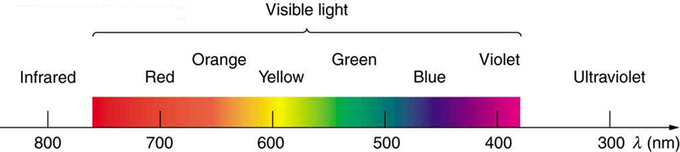Colors of a Rainbow: Even though rainbows are associated with seven colors, the rainbow is a continuous distribution of colors according to wavelengths.

Refraction is responsible for dispersion in rainbows and many other situations. The angle of refraction depends on the index of refraction, as we saw in the Law of Refraction. We know that the index of refraction n depends on the medium. But for a given medium, n also depends on wavelength. Note that, for a given medium, n increases as wavelength decreases and is greatest for violet light. Thus violet light is bent more than red light and the light is dispersed into the same sequence of wavelengths.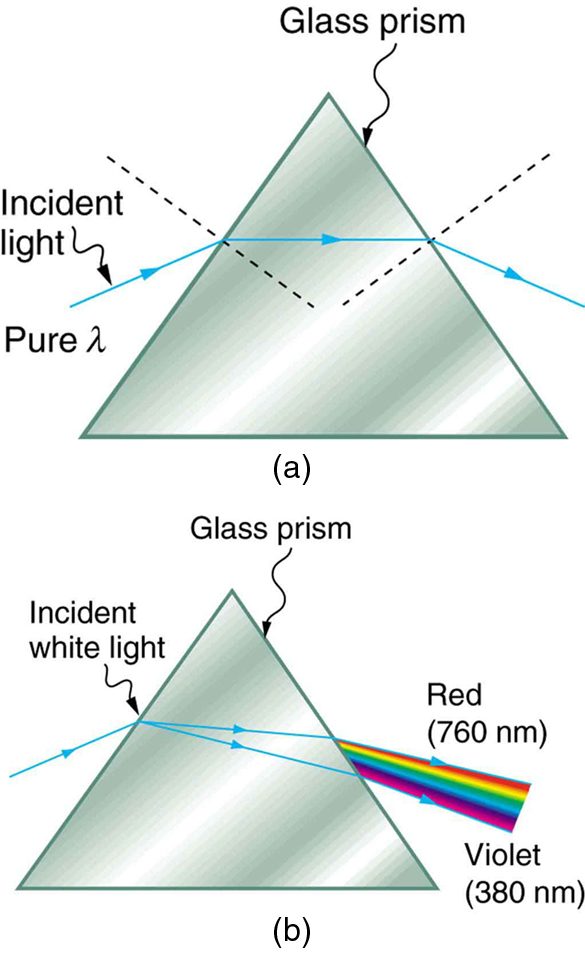Pure Light and Light Dispersion: (a) A pure wavelength of light falls onto a prism and is refracted at both surfaces. (b) White light is dispersed by the prism (shown exaggerated). Since the index of refraction varies with wavelength, the angles of refraction vary with wavelength. A sequence of red to violet is produced, because the index of refraction increases steadily with decreasing wavelength.

Rainbows are produced by a combination of refraction and reflection. You may have noticed that you see a rainbow only when you look away from the sun. Light enters a drop of water and is reflected from the back of the drop. The light is refracted both as it enters and as it leaves the drop. Since the index of refraction of water varies with wavelength, the light is dispersed, and a rainbow is observed. (There is no dispersion caused by reflection at the back surface, since the law of reflection does not depend on wavelength. ) The actual rainbow of colors seen by an observer depends on the myriad of rays being refracted and reflected toward the observer’s eyes from numerous drops of water. The arc of a rainbow comes from the need to be looking at a specific angle relative to the direction of the sun.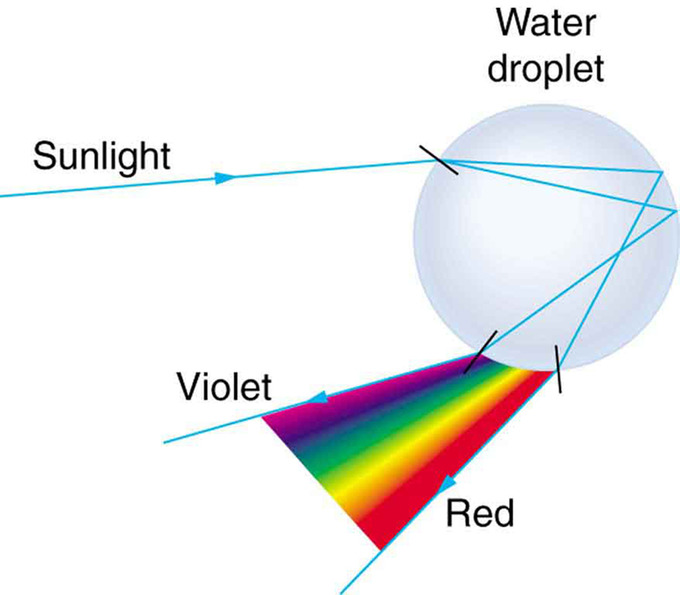Light Reflecting on Water Droplet: Part of the light falling on this water drop enters and is reflected from the back of the drop. This light is refracted and dispersed both as it enters and as it leaves the drop.

## Thin Lenses and Ray Tracing

Ray tracing is the technique of determining the paths light rays take; often thin lenses (the light ray bending only once) are assumed.

### LEARNING OBJECTIVES

Describe properties of a thin lens and the purpose of ray tracing

### KEY TAKEAWAYS

#### Key Points

• When light interacts with objects several times as large as its wavelength, it travels in straight lines and acts like a ray. A ray is simply a straight line that originates at a point.
• Ray tracing is the method for determining the paths light takes through matter, such as optical systems that include lenses.
• A thin lens is defined as one with a thickness that allows rays to refract, as illustrated in, but that does not allow properties such as dispersion and aberrations. An ideal thin lens has two refracting surfaces but the lens is thin enough to assume that light rays bend only once.
• There are five basic rules for tracing rays through a lens.

#### Key Terms

• focal point: A focus—a point at which rays of light or other radiation converge.
• ray tracing: A technique used in optics for analysis of optical systems.
• thin lens: A thin lens is defined to be one whose thickness allows rays to refract but does not allow properties such as dispersion and aberrations.

### Thin Lenses and Ray Tracing

Ray tracing is the technique of determining or following (tracing) the paths that light rays take. Experiments, as well as our own experiences, show that when light interacts with objects several times as large as its wavelength, it travels in straight lines and acts like a ray. (A ray is simply a straight line that originates at a point. ) Its wave characteristics are not pronounced in such situations. Since the wavelength of light is less than a micron (a thousandth of a millimeter), it acts like a ray in the many common situations in which it encounters objects larger than a micron, such as lenses.

For rays passing through matter, the law of refraction is used to trace the paths. Here we use ray tracing to help us understand the action of lenses in situations ranging from forming images on film to magnifying small print to correcting nearsightedness. While ray tracing for complicated lenses, such as those found in sophisticated cameras, may require computer techniques, there is a set of simple rules for tracing rays through thin lenses. A thin lens is defined to be one whose thickness allows rays to refract, as illustrated in, but does not allow properties such as dispersion and aberrations. An ideal thin lens has two refracting surfaces but the lens is thin enough toassume that light rays bend only once. Another way of saying this is that the lens thickness is much much smaller than the focal length of the lens. A thin symmetrical lens has two focal points, one on either side and both at the same distance from the lens. (See. ) Another important characteristic of a thin lens is that light rays through its center are deflected by a negligible amount, as seen in the center rays in the first two figures. The treatment of a lens as a thin lens is known as the “thin lens approximation. ”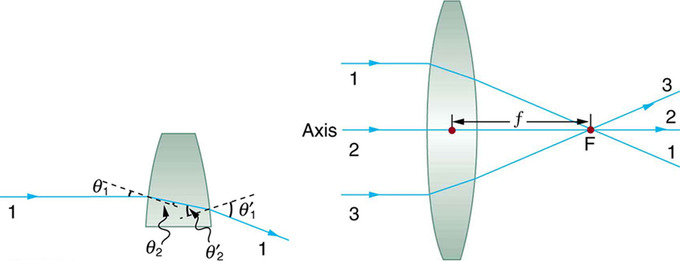Convex Lens: Rays of light entering a converging lens parallel to its axis converge at its focal point F. (Ray 2 lies on the axis of the lens. ) The distance from the center of the lens to the focal point is the lens’s focal length f. An expanded view of the path taken by ray 1 shows the perpendiculars and the angles of incidence and refraction at both surfaces.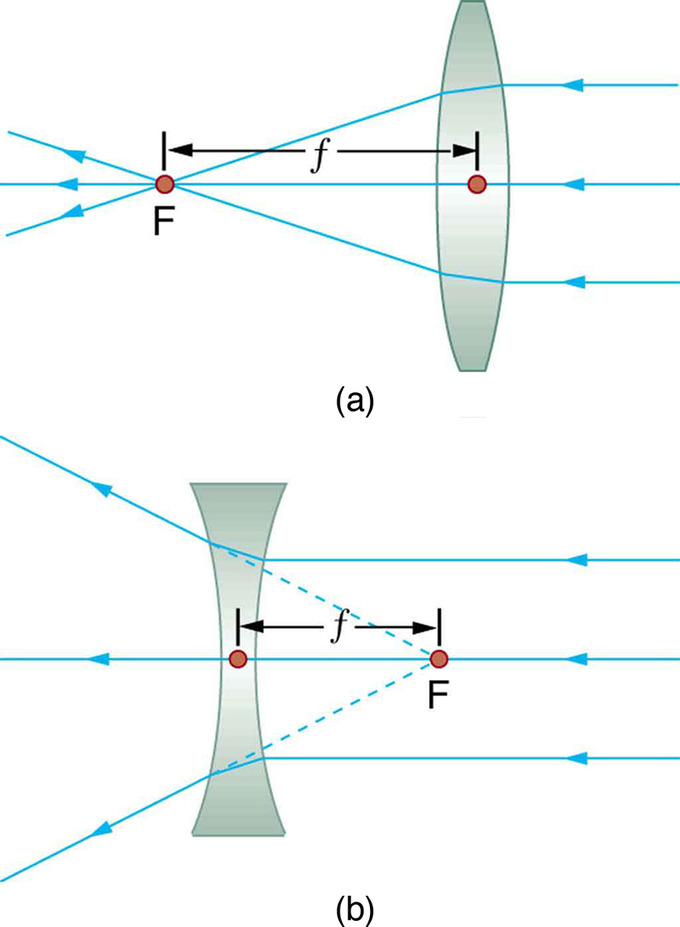Thin Lens: Thin lenses have the same focal length on either side. (a) Parallel light rays entering a converging lens from the right cross at its focal point on the left. (b) Parallel light rays entering a diverging lens from the right seem to come from the focal point on the right.

### Rules for Ray Tracing

Using paper, pencil, and a straight edge, ray tracing can accurately describe the operation of a lens. The rules for ray tracing for thin lenses are based on the illustrations included in this section:

1. A ray entering a converging lens parallel to its axis passes through the focal point F of the lens on the other side. ( See rays 1 and 3 in. )
2. A ray entering a diverging lens parallel to its axis seems to come from the focal point F. (See rays 1 and 3 in. )
3. A ray passing through the center of either a converging or a diverging lens does not change direction. (See ray 2 in and. )
4. A ray entering a converging lens through its focal point exits parallel to its axis. (The reverse of rays 1 and 3 in. )
5. A ray that enters a diverging lens by heading toward the focal point on the opposite side exits parallel to the axis. (The reverse of rays 1 and 3 in ).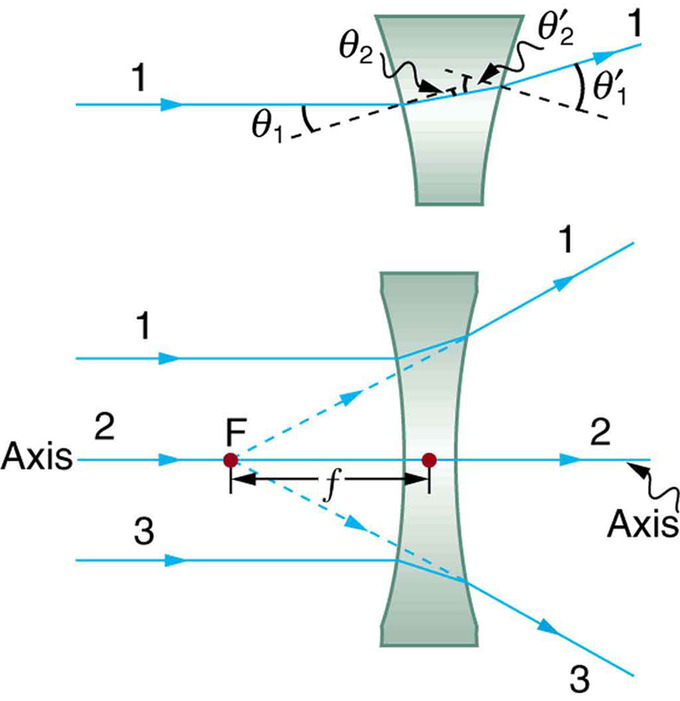Diverging Lens: Rays of light entering a diverging lens parallel to its axis are diverged, and all appear to originate at its focal point F. The dashed lines are not rays—they indicate the directions from which the rays appear to come. The focal length f of a diverging lens is negative. An expanded view of the path taken by ray 1 shows the perpendiculars and the angles of incidence and refraction at both surfaces.

Ray Diagrams, Concave Lens and Convex Mirror: Shows how to draw the ray diagrams for locating the image produced by a concave lens and a convex mirror.

## The Thin Lens Equation and Magnification

The thin lens equation relates the object distance do, image distance di, and focal length f.

### LEARNING OBJECTIVES

Formulate five basic rules of ray tracing

### KEY TAKEAWAYS

#### Key Points

• Ray tracing can be used to construct an image from the light rays originating from an object that pass through a lens. The image is located at the point where the rays cross. By choosing several points from an object the entire image can be constructed.
• We define do to be the object distance, the distance of an object from the center of a lens. Image distance di is defined to be the distance of the image from the center of a lens. The height of the object and height of the image are given the symbols ho and hi, respectively.
• The thin lens equation quickly provides the relation between di, do, and the focal length f. It can be derived from a geometric analysis of ray tracing for thin lenses and is given by
$\frac{1}{{\text{d}}_{\text{o}}}+\frac{1}{{\text{d}}_{\text{i}}}=\frac{1}{\text{f}}$

.

• The magnification m of an image is the ratio between the image and object height (hi/ho). The magnification is related to do, di, ho, and hi by the following relation:
$\frac{{\text{h}}_{\text{i}}}{{\text{h}}_{\text{o}}}=-\frac{{\text{d}}_{\text{i}}}{{\text{d}}_{\text{o}}}=\text{m}$

.

#### Key Terms

• thin lens equation: Relates object distance do, image distance di, and focal length f:
$\frac{1}{{\text{d}}_{\text{o}}}+\frac{1}{{\text{d}}_{\text{i}}}=\frac{1}{\text{f}}$

• image distance: The distance of the image from the center of the lens.
• magnification: The apparent enlargement of an object in an image.

### Image Formation by Thin Lenses

How does a lens form an image of an object? We can use the technique of ray tracing to illustrate how lenses form images. We can also develop equations to describe the images quantitatively. Recall the five basic rules of ray tracing:

1. A ray entering a converging lens parallel to its axis passes through the focal point F of the lens on the other side.
2. A ray entering a diverging lens parallel to its axis seems to come from the focal point F.
3. A ray passing through the center of either a converging or a diverging lens does not change direction.
4. A ray entering a converging lens through its focal point exits parallel to its axis.
5. A ray that enters a diverging lens by heading toward the focal point on the opposite side exits parallel to the axis.

Consider an object some distance away from a converging lens, as shown in. To find the location and size of the image formed, we trace the paths of selected light rays originating from one point on the object (in this case the top of the person’s head). The figure shows three rays from the top of the object that can be traced using the five ray tracing rules. Rays leave this point going in many directions, but we concentrate on only a few with paths that are easy to trace. The first ray is one that enters the lens parallel to its axis and passes through the focal point on the other side (rule 1). The second ray passes through the center of the lens without changing direction (rule 3). The third ray passes through the nearer focal point on its way into the lens and leaves the lens parallel to its axis (rule 4). The three rays cross at the same point on the other side of the lens. The image of the top of the person’s head is located at this point. All rays that come from the same point on the top of the person’s head are refracted in such a way as to cross at the point shown. Rays from another point on the object, such as her belt buckle, will also cross at another common point, forming a complete image, as shown. Although three rays are traced in, only two are necessary to locate the image. It is best to trace rays for which there are simple ray tracing rules. Before applying ray tracing to other situations, let us consider the example shown in in more detail.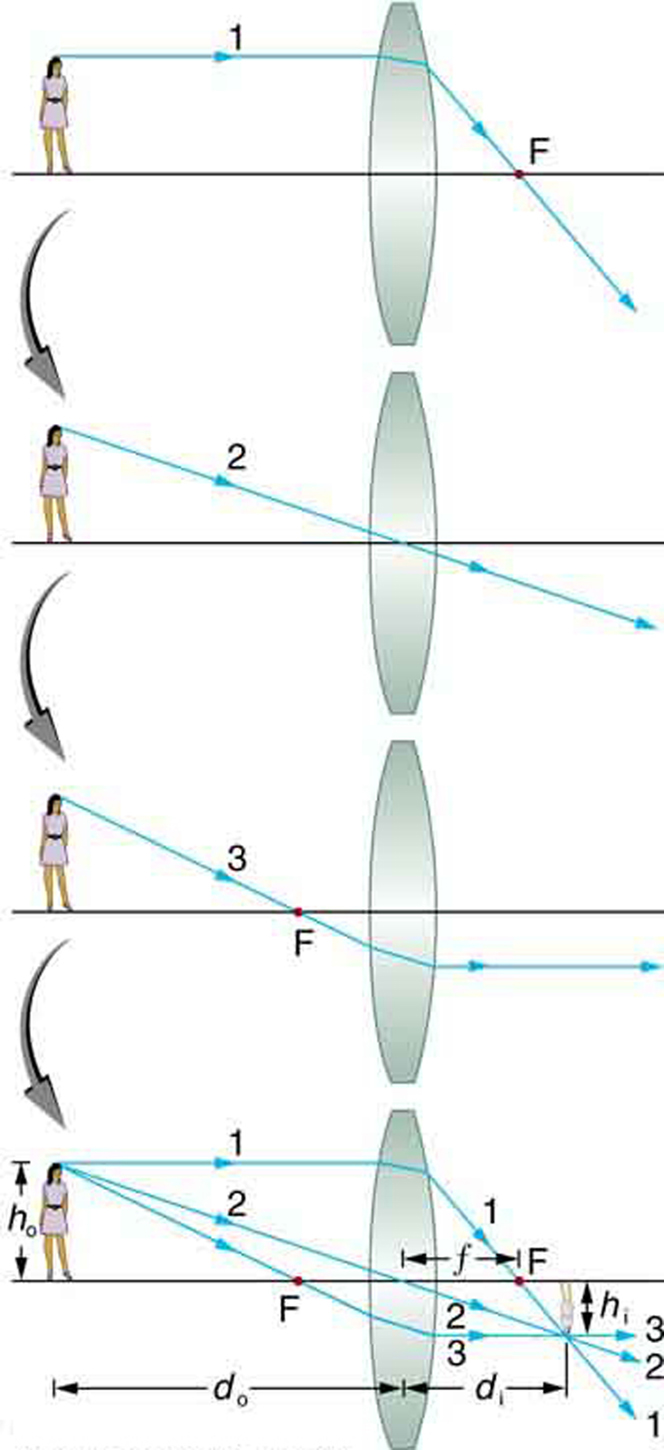Image Formation with a Thin Lens: Ray tracing is used to locate the image formed by a lens. Rays originating from the same point on the object are traced—the three chosen rays each follow one of the rules for ray tracing, so that their paths are easy to determine. The image is located at the point where the rays cross. In this case, a real image—one that can be projected on a screen—is formed.

Several important distances appear in. We define do as the object distance—the distance of an object from the center of a lens. Image distance diis defined as the distance of the image from the center of a lens. The height of the object and height of the image are given the symbols ho and hi, respectively. Images that appear upright relative to the object have heights that are positive and those that are inverted have negative heights. Using the rules of ray tracing and making a scale drawing with paper and pencil, like that in, we can accurately describe the location and size of an image. But the real benefit of ray tracing is in visualizing how images are formed in a variety of situations. To obtain numerical information, we use a pair of equations that can be derived from a geometric analysis of ray tracing for thin lenses. The thin lens equation is:

$\frac{1}{{\text{d}}_{\text{o}}}+\frac{1}{{\text{d}}_{\text{i}}}=\frac{1}{\text{f}}$

We define the ratio of image height to object height (hi/ho) as the magnification m. The magnification is related to do, di, ho, and hi by the following relation:

$\frac{{\text{h}}_{\text{i}}}{{\text{h}}_{\text{o}}}=-\frac{{\text{d}}_{\text{i}}}{{\text{d}}_{\text{o}}}=\text{m}$

In many cases both of these equations are referred to together as the thin lens equations. The thin lens equations are broadly applicable to all situations involving thin lenses (and “thin” mirrors).

Thin Lens Equations for a Convex Lens: Shows how to use the thin lens equation to calculate the image distance, image height and image orientation for convex lenses when the object distance is greater the the focal length (f).

## Combinations of Lenses

A compound lens is an array of simple lenses with a common axis.

### LEARNING OBJECTIVES

Calculate focal length for a compound lens from the focal lengths of simple lenses

### KEY TAKEAWAYS

#### Key Points

• The use of multiple elements allows for the correction of more optical aberrations, such as the chromatic abberation caused by the wavelength -dependent index of refraction in glass, than is possible using a single lens.
• If the lenses of focal lengths f1 and f2are “thin”, the combined focal length f of the lenses is given by
$\frac{1}{\text{f}}=\frac{1}{{\text{f}}_{1}}+\frac{1}{{\text{f}}_{2}}$

while if the lenses are separated by some distance d then the combined focal length is given by

$\frac{1}{\text{f}}=\frac{1}{{\text{f}}_{1}}+\frac{1}{{\text{f}}_{2}}-\frac{\text{d}}{{\text{f}}_{1}{\text{f}}_{2}}$

.

• If the separation distance is equal to the sum of the focal lengths (d = f1+f2), the combined focal length is infinite. This corresponds to a pair of lenses that transform a collimated beam into another collimated beam. This type of system is called an afocal system (a simple optical telescope).
• An achromatic doublet is a kind of compound lens designed to bring two wavelengths (typically red and blue/violet) into focus in the same plane. This (partially) corrects for the chromatic aberration found in a single simple lens. See.

#### Key Terms

• aberration: The convergence to different foci, by a lens or mirror, of rays of light emanating from one and the same point, or the deviation of such rays from a single focus; a defect in a focusing mechanism that prevents the intended focal point.
• afocal system: An optical system that produces no net convergence or divergence of the beam, i.e. has an infinite effective focal length. This type of system can be created with a pair of optical elements where the distance between the elements is equal to the sum of each element’s focal length (d = f1+f2).
• achromatic doublet: A type of lens made up of two simple lenses paired together designed so that the chromatic aberration of each lens partially offsets the other; in this way light in a range of wavelengths may be brought to the same focus.

### COMPOUND LENSES

In contrast to a simple lens, which consists of only one optical element, a compound lens is an array of simple lenses (elements) with a common axis. The use of multiple elements allows for the correction of more optical aberrations, such as the chromatic aberration caused by the wavelength-dependent index of refraction in glass, than is possible using a single lens. In many cases these aberrations can be compensated for to a great extent by using a combination of simple lenses with complementary aberrations.

The simplest case is where lenses are placed in contact: if the lenses of focal lengths f1 and f2 are “thin”, the combined focal length f of the lenses is given by

$\frac{1}{\text{f}}=\frac{1}{{\text{f}}_{1}}+\frac{1}{{\text{f}}_{2}}$

.

Since 1/f is the power of a lens, it can be seen that the powers of thin lenses in contact are additive.

If two thin lenses are separated in air by some distance d (where d is smaller than the focal length of the first lens), the focal length for the combined system is given by

$\frac{1}{\text{f}}=\frac{1}{{\text{f}}_{1}}+\frac{1}{{\text{f}}_{2}}-\frac{\text{d}}{{\text{f}}_{1}{\text{f}}_{2}}$

.

### BACK FOCAL LENGTH

The distance from the second lens to the focal point of the combined lenses is called the back focal length (BFL).

$\text{BFL}=\frac{{\text{f}}_{2}\left(\text{d}-{\text{f}}_{1}\right)}{\text{d}-\left({\text{f}}_{1}+{\text{f}}_{2}\right)}$

.

As d tends to zero, the value of the BFL tends to the value of f given for thin lenses in contact.

If the separation distance is equal to the sum of the focal lengths (d = f1+f2), the combined focal length and BFL are infinite. This corresponds to a pair of lenses that transform a parallel (collimated) beam into another collimated beam (see ). This type of system is called an afocal system, since it produces no net convergence or divergence of the beam. Two lenses at this separation form the simplest type of optical telescope. Although the system does not alter the divergence of a collimated beam, it does alter the width of the beam. The magnification of such a telescope is given by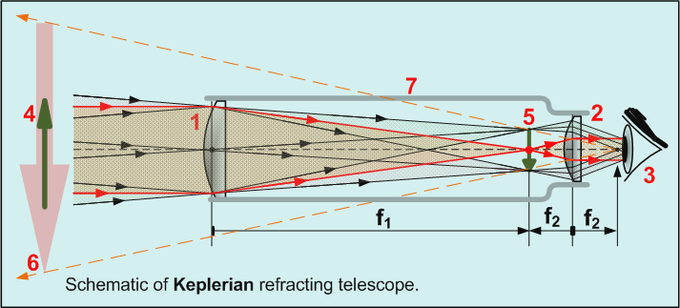Keplerian Telescope: All refracting telescopes use the same principles. The combination of an objective lens 1 and some type of eyepiece 2 is used to gather more light than the human eye could collect on its own, focus it 5, and present the viewer with a brighter, clearer, and magnified virtual image 6. The magnification can be found by dividing the focal length of the objective lens by the focal length of the eyepiece.

$\text{M}=-\frac{{\text{f}}_{2}}{{\text{f}}_{1}}$

which is the ratio of the input beam width to the output beam width. Note the sign convention: a telescope with two convex lenses (f1 > 0, f2 > 0) produces a negative magnification, indicating an inverted image. A convex plus a concave lens (f1 > 0 >f2) produces a positive magnification and the image is upright.

### ACHROMATS

An achromatic lens or achromat is a lens that is designed to limit the effects of chromatic and spherical aberration. Achromatic lenses are corrected to bring two wavelengths (typically red and blue/violet) into focus in the same plane.

The most common type of achromat is the achromatic doublet, which is composed of two individual lenses made from glasses with different amounts of dispersion Typically, one element is a negative (concave) element made out of flint, which has relatively high dispersion, and the other is a positive (convex) element made of crown glass, which has lower dispersion. The lens elements are mounted next to each other, often cemented together, and shaped so that the chromatic aberration of one is counterbalanced by that of the other.

In the most common type (shown in ), the positive power of the crown lens element is not quite equaled by the negative power of the flint lens element. Together they form a weak positive lens that will bring two different wavelengths of light to a common focus. Negative doublets, in which the negative-power element predominates, are also made.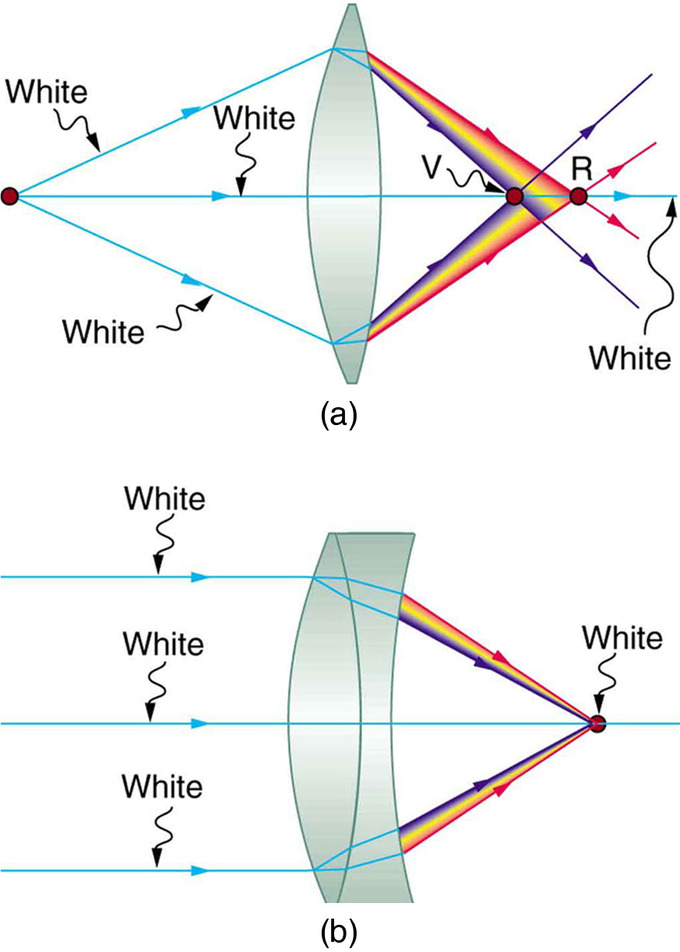Achromatic Doublet: (a) Chromatic aberration is caused by the dependence of a lens’s index of refraction on color (wavelength). The lens is more powerful for violet (V) than for red (R), producing images with different locations and magnifications. (b) Multiple-lens systems, such as this achromatic doublet, can partially correct chromatic aberrations, but they may require lenses of different materials and add to the expense of optical systems such as cameras.

## The Lensmaker’s Equation

The lensmaker’s formula is used to relate the radii of curvature, the thickness, the refractive index, and the focal length of a thick lens.

### LEARNING OBJECTIVES

Compare idealized thin lenses with real lenses

### KEY TAKEAWAYS

#### Key Points

• If a lens is biconvex, a beam of light travelling parallel to the lens axis and passing through the lens will be focused to a spot on the axis, at a certain distance behind the lens (i.e. the focal length ). In this case, the lens is called a positive or converging lens. See.
• If a lens is biconcave, a beam of light passing through the lens is diverged (spread); the lens is thus called a negative or diverging lens. See.
• The focal length of a thick lens in air can be calculated from the lensmaker’s equation:
$\text{P}=\frac{1}{\text{f}}=\left(\text{n}-1\right)\left[\frac{1}{{\text{R}}_{1}}-\frac{1}{{\text{R}}_{2}}+\frac{\left(\text{n}-1\right)\text{d}}{\text{n}{\text{R}}_{1}{\text{R}}_{2}}\right]$

.

• The signs of the lens’ radii of curvature indicate whether the corresponding surfaces are convex or concave. The signs are reversed for the back surface of the lens: if R2 is positive the surface is concave, and if R2 is negative the surface is convex.
• The lensmaker’s equation can be greatly simplified if the lens thickness d is very small compared to R1 and R2. In this case, the thin lens approximation can then be made and the lensmaker’s equation can be approximated as
$\text{P}=\frac{1}{\text{f}}\approx \left(\text{n}-1\right)\left[\frac{1}{{\text{R}}_{1}}-\frac{1}{{\text{R}}_{2}}\right]$

.

#### Key Terms

• thick lens: Lenses whose thicknesses are not negligible (i.e., one cannot make the simple assumption that a light ray is refracted only once in the lens).
• surface vertices: The points where each surface crosses the optical axis. They are important primarily because they are the physically measurable parameters for the position of the optical elements, and so the positions of the other cardinal points must be known with respect to the vertices to describe the physical system.

### Thick Lenses

Unlike idealized thin lenses, real lenses have a finite thickness between their two surfaces of curvature. An ideal thin lens with two surfaces of equal curvature would have zero optical power, meaning that it would neither converge nor diverge light. A lens whose thickness is not negligible is called a thick lens. In this case, we can not simply assume that a light ray is only refracted once while traveling through the lens. Instead the extent of the refraction must be dependent on the thickness of the lens.

Lenses are classified by the curvature of the two optical surfaces. A lens is biconvex (or double convex, or just convex) if both surfaces are convex. If the lens is biconvex, a beam of light travelling parallel to the lens axis and passing through the lens will be converged (or focused) to a spot on the axis, at a certain distance behind the lens (i.e. the focal length). In this case, the lens is called a positive or converging lens. See for a diagram of a positive (converging) lens.Thick Converging Lens: Diagram of a positive (converging) lens. The lensmaker’s formula relates the radii of curvature, the index of refraction of the lens, the thickness of the lens, and the focal length.

If the lens is biconcave, a beam of light passing through the lens is diverged (spread); the lens is thus called a negative or diverging lens. The beam after passing through the lens appears to be emanating from a particular point on the axis in front of the lens; the distance from this point to the lens is also known as the focal length, although it is negative with respect to the focal length of a converging lens. See for a diagram of a negative (diverging) lens.Negative Diverging Lens: Diagram of a negative (diverging) lens. The lensmaker’s formula relates the radii of curvature, the index of refraction of the lens, the thickness of the lens, and the focal length.

The focal length of a thick lens in air can be calculated from the lensmaker’s equation:

$\text{P}=\frac{1}{\text{f}}=\left(\text{n}-1\right)\left[\frac{1}{{\text{R}}_{1}}-\frac{1}{{\text{R}}_{2}}+\frac{\left(\text{n}-1\right)\text{d}}{\text{n}{\text{R}}_{1}{\text{R}}_{2}}\right]$

where

• P is the power of the lens,
• f is the focal length of the lens,
• n is the refractive index of the lens material,
• R1is the radius of curvature of the lens surface closest to the light source,
• R2 is the radius of curvature of the lens surface farthest from the light source, d and is the thickness of the lens (the distance along the lens axis between the two surface vertices).

### Sign convention of Radii R1 and R2

The signs of the lens’ radii of curvature indicate whether the corresponding surfaces are convex or concave. The sign convention used to represent this varies, but for our treatment if R1 is positive the first surface is convex, and if R1 is negative the surface is concave. The signs are reversed for the back surface of the lens: if R2 is positive the surface is concave, and if R2 is negative the surface is convex. If either radius is infinite, the corresponding surface is flat. With this convention the signs are determined by the shapes of the lens surfaces, and are independent of the direction in which light travels through the lens.

### Thin Lens Approximation

The above equation can be greatly simplified if the lens thickness d is very small compared to R1 and R2. In this case, the thin lens approximation can then be made and the lensmaker’s equation can be approximated as

$\text{P}=\frac{1}{\text{f}}\approx \left(\text{n}-1\right)\left[\frac{1}{{\text{R}}_{1}}-\frac{1}{{\text{R}}_{2}}\right]$

The focal length f is positive for converging lenses, and negative for diverging lenses. The reciprocal of the focal length, 1/f, is the optical of the lens. If the focal length is in meters, this gives the optical power in diopters (inverse meters).

Lenses have the same focal length when light travels from the back to the front as when light goes from the front to the back, although other properties of the lens, such as the aberrations are not necessarily the same in both directions.

## Refraction Through Lenses

Because the index of refraction of a lens is greater than air, a ray moves towards the perpendicular as it enters and away as it leaves.

### LEARNING OBJECTIVES

Compare the effect of a convex lens and a concave lens on the light rays

### KEY TAKEAWAYS

#### Key Points

• Recall that the a ray will bend as it enters a medium with a different refractive index. Since the refractive index of a lens is greater than air, a light ray will move towards the perpendicular as it enters and away as it leaves.
• A convex lens has been shaped so that all light rays that enter it parallel to its axis cross one another at a single point on the opposite side of the lens (the focal point ). Such a lens is called a converging (or convex) lens for the converging effect it has on light rays. See.
• A concave lens is a diverging lens, because it causes the light rays to bend away (diverge) from its axis. shows the effect it has on rays of light that enter it parallel to its axis (the path taken by ray 2 in the figure is the axis of the lens).
• The greater effect a lens has on light rays, the more powerful it is said to be. A powerful converging lens will focus parallel light rays closer to itself and will have a smaller focal length than a weak lens. The power of a lens is given by the equation
$\text{P}=\frac{1}{\text{f}}$

.

#### Key Terms

• convex lens: A lens having at least one convex surface, such that light passing through it, may be brought to a focus.
• concave lens: A lens having at least one concave surface, such that light rays passing through it bend away from its optical axis.
• focal point: A focus—a point at which rays of light or other radiation converge.

### Refraction Through Lenses

Lenses are found in a huge array of optical instruments, ranging from the simple magnifying glass to a camera lens to the lens of the human eye. The word lens derives from the Latin word for lentil bean—the shape of which is similar to that of the convex lens (as shown in ). The convex lens is shaped so that all light rays that enter it parallel to its axis cross one another at a single point on the opposite side of the lens. The axis is defined as a line normal to the lens at its center (as shown in ). Such a lens is called a converging (or convex) lens for the corresponding effect it has on light rays. The expanded view of the path of one ray through the lens illustrates how the ray changes direction both as it enters and as it leaves the lens.

Since the index of refraction of the lens is greater than that of air, the ray moves towards the perpendicular as it enters, and away from the perpendicular as it leaves (this is in accordance with the law of refraction). Due to the lens’s shape, light is thus bent toward the axis at both surfaces. The point at which the rays cross is defined as the focal point F of the lens. The distance from the center of the lens to its focal point is defined as the focal length f of the lens. shows how a converging lens, such as that in a magnifying glass, can concentrate (converge) the nearly parallel light rays from the sun towards a small spot.Magnifying Glass: Sunlight focused by a converging magnifying glass can burn paper. Light rays from the sun are nearly parallel and cross at the focal point of the lens. The more powerful the lens, the closer to the lens the rays will cross.

The greater effect a lens has on light rays, the more powerful it is said to be. For example, a powerful converging lens will focus parallel light rays closer to itself and will have a smaller focal length than a weak lens. The light will also focus into a smaller, more intense spot for a more powerful lens. The power P of a lens is defined as the inverse of its focal length. In equation form:

$\text{P}=\frac{1}{\text{f}}$

shows the effect of a concave lens on rays of light entering it parallel to its axis (the path taken by ray 2 in the figure is the axis of the lens). The concave lens is a diverging lens, because it causes the light rays to bend away (diverge) from its axis. In this case, the lens is shaped so that all light rays entering it parallel to its axis appear to originate from the same point F, defined as the focal point of a diverging lens. The distance from the center of the lens to the focal point is again called the focal length f of the lens. Note that the focal length and power of a diverging lens are defined as negative. For example, if the distance to F in is 5.00 cm, then the focal length is f=–5.00 cm and the power of the lens is P=–20 D. The expanded view of the path of one ray through the lens illustrates how the shape of the lens (given the law of refraction) causes the ray to follow its particular path and be diverged.

In subsequent sections we will examine the technique of ray tracing to describe the formation of images by lenses. Additionally, we will explore how image locations and characteristics can be quantified with the help of a set of geometric optics equations.

## Image Reflection by a Plane Mirror

A mirror is a reflective surface that bounces off light, thus producing a real or virtual image.

### LEARNING OBJECTIVES

Describe interaction of the light with a mirror surface

### KEY TAKEAWAYS

#### Key Points

• Reflected images can be either real or virtual. In a plane mirror, the images are virtual.
• The virtual images in a plane mirror have a left-right inversion.
• Drawing a ray diagram is a way to predict what a reflected image will look like.

#### Key Terms

• virtual image: A virtual image occurs when light rays do not actually meet at the image

### Plane Mirrors and Reflection

A mirror is a reflective surface that does not allow the passage of light and instead bounces it off, thus producing an image. The most common mirrors are flat and called plane mirrors. These mirrors are made by putting a thin layer of silver nitrate or aluminium behind a flat piece of glass.

When you place an object in front of a mirror, you see an image of the same object in the mirror. The object is the source of the incident rays, and the image is formed by the reflected rays. An image formed by reflection may be real or virtual. A “real” image occurs when light rays actually intersect at the image, and become inverted, or turned upside down. A “virtual” image occurs when light rays do not actually meet at the image. Instead, you “see” the image because your eye projects light rays backward. You are fooled into seeing an image! A virtual image is right side up (upright).

In flat, or plane mirrors, the image is a virtual image, and is the same distance behind the mirror as the object is in front of the mirror. The image is also the same size as the object. These images are also parity inverted, which means they have a left-right inversion.

### Ray Diagrams

The way that we can predict how a reflection will look is by drawing a ray diagram. These diagrams can be used to find the position and size of the image and whether that image is real or virtual. These are the steps you follow to draw a ray diagram:

1. Draw the plane mirror as a straight line on a principal axis. The principal axis is an imaginary line that is drawn perpendicular to the mirror.
2. Draw the object as an arrow in front of the mirror.
3. Draw the image of the object, by using the principle that the image is placed at the same distance behind the mirror that the object is in front of the mirror. The image size is also the same as the object size. shows these first three steps.
4. Place a dot at the point the eye is located.
5. Pick one point on the image and draw the reflected ray that travels to the eye as it sees this point. Remember to add an arrowhead.
6. Draw the incident ray for light traveling from the corresponding point on the object to the mirror, such that the law of reflection is obeyed.
7. Continue for other extreme points on the object (i.e. the tip and base of the arrow). A completed ray diagram is shown in

The angle in which a light ray hits the mirror is the same angle in which it will be reflected back. If, for example, a light ray leaves the top of an object travelling parallel to the principal axis, it will hit the mirror at a 0 degree angle, and be reflected back at 0 degrees. When this happens, we say the ray hit the mirror normally. If the light ray hit the object at a 30 degree angle, it will be reflected back at a 30 degree angle.

## Image Formation by Spherical Mirrors: Reflection and Sign Conventions

A mirror is a reflective surface that light does not pass through, made by a layer of silver nitrate or aluminium behind piece of glass.

### LEARNING OBJECTIVES

Distinguish properties of the concave and the convex mirrors

### KEY TAKEAWAYS

#### Key Points

• Images in mirrors can be either real or virtual.
• A summary of the properties of the concave mirrors are shown below: converging real image inverted image in front of mirror.
• A summary of the properties of the convex mirrors are shown below: diverging virtual image upright image behind mirror.

#### Key Terms

• concave: curved like the inner surface of a sphere or bowl
• convex: curved or bowed outward like the outside of a bowl or sphere or circle

### Overview

A mirror is a reflective surface that light does not pass through, but bounces off of and this produces an image. Mirrors are made by putting a thin layer of silver nitrate or aluminium behind a flat piece of glass.

When you place an object in front of a mirror, you see the same object in the mirror. This image that appears to be behind the mirror is called the image. The object is the source of the incident rays, and the image is formed by the reflected rays. An image formed by reflection may be real or virtual. A real image occurs when light rays actually intersect at the image, and is inverted, or upside down. A virtual image occurs when light rays do not actually meet at the image. Instead, you “see” the image because your eye projects light rays backward. A virtual image is right side up (upright).

This section will cover spherical mirrors. Spherical mirrors can be either concave or convex. The center of curvature is the point at the center of the sphere and describes how big the sphere is. These concepts are shown in.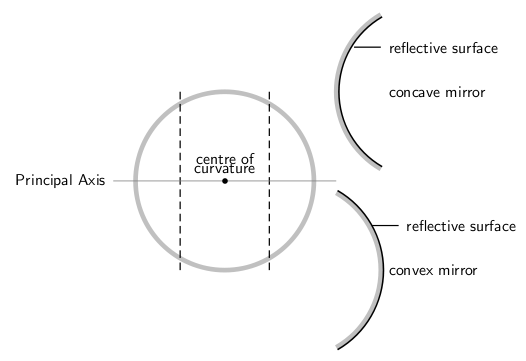Spherical Mirrors: This figure shows the difference between a concave and convex mirror.

### Concave Mirrors

In a concave mirror, the principal axis is a line that is perpendicular to the center of the mirror. The easiest way to visualize what a image will look like in this type of mirror is a ray diagram. Before that can be done, the focal point must first be defined. This point is half way between the mirror and the center of curvature on the principal axis. The distance to the focal point from the mirror is called the focal length. We can see from the figure that this focal length is also equal to half of the radius of the curvature. shows the ray diagram of a concave mirror.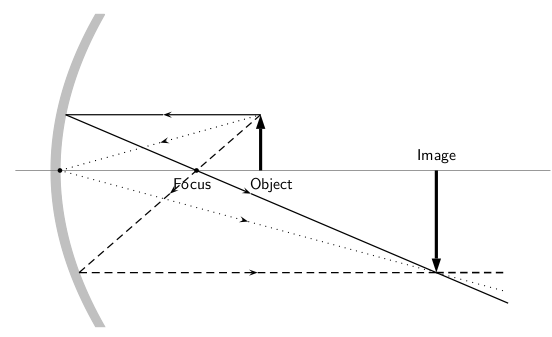Concave Ray Diagram: This is a ray diagram of a concave mirror. The steps taken to draw are the same as those in a plane mirror.

A summary of the properties of concave mirrors is shown below:

• converging
• real image
• inverted
• image in front of mirror

### Convex Mirrors

In convex mirrors, the principal axis is the same as in a plane or concave mirror, perpendicular to the center of the mirror. In this case, the focal point is behind the mirror. A convex mirror has a negative focal length because of this. The focal point is the same distance from the mirror as in a concave mirror. This is shown in.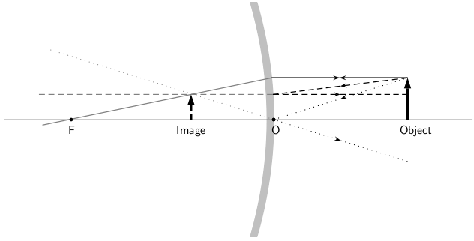Convex Mirror Ray Diagram: A convex mirror with three rays drawn to locate the image. Each incident ray is reflected according to the Law of Reflection. The reflected rays diverge. If the reflected rays are extended behind the mirror, then their intersection gives the location of the image behind the mirror. For a convex mirror, the image is virtual and upright.

A summary of the properties of convex mirrors is shown below:

• diverging
• virtual image
• upright
• image behind mirror

Billing Information